# TwoPlug

Component with one polyphase electrical port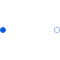# Information

This information is part of the Modelica Standard Library maintained by the Modelica Association.

Superclass of elements which have two electrical plugs: the positive plug connector plug_p, and the negative plug connector plug_n. The currents flowing into plug_p are provided explicitly as currents i[m].

# Connectors (2)

plug_p plug_n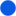Type: PositivePlug Description: Positive polyphase electrical plug with m pins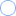Type: NegativePlug Description: Negative polyphase electrical plug with m pins

# Extended by (28)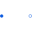OnePort Modelica.Electrical.MultiPhase.Interfaces Component with two electrical plugs and currents from plug_p to plug_n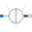CosineCurrent Modelica.Electrical.MultiPhase.Sources Multiphase cosine current source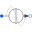SineCurrent Modelica.Electrical.MultiPhase.Sources Multiphase sine current source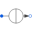ConstantCurrent Modelica.Electrical.MultiPhase.Sources Multiphase constant current source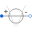CosineVoltage Modelica.Electrical.MultiPhase.Sources Multiphase cosine voltage source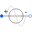SineVoltage Modelica.Electrical.MultiPhase.Sources Multiphase sine voltage source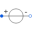ConstantVoltage Modelica.Electrical.MultiPhase.Sources Multiphase constant voltage source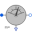CurrentQuasiRMSSensor Modelica.Electrical.MultiPhase.Sensors Continuous quasi current RMS sensor for multi phase systemVoltageQuasiRMSSensor Modelica.Electrical.MultiPhase.Sensors Continuous quasi voltage RMS sensor for multi phase system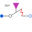CloserWithArc Modelica.Electrical.MultiPhase.Ideal Multiphase closer with arc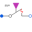OpenerWithArc Modelica.Electrical.MultiPhase.Ideal Multiphase opener with arc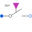IdealClosingSwitch Modelica.Electrical.MultiPhase.Ideal Multiphase ideal closer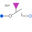IdealOpeningSwitch Modelica.Electrical.MultiPhase.Ideal Multiphase ideal opener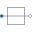Short Modelica.Electrical.MultiPhase.Ideal Multiphase short cut branch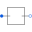Idle Modelica.Electrical.MultiPhase.Ideal Multiphase idle branch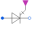IdealGTOThyristor Modelica.Electrical.MultiPhase.Ideal Multiphase ideal GTO thyristorIdealThyristor Modelica.Electrical.MultiPhase.Ideal Multiphase ideal thyristor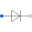IdealDiode Modelica.Electrical.MultiPhase.Ideal Multiphase ideal diode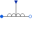VariableInductor Modelica.Electrical.MultiPhase.Basic Ideal linear electrical inductors with variable inductance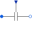VariableCapacitor Modelica.Electrical.MultiPhase.Basic Ideal linear electrical capacitors with variable capacitance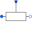VariableConductor Modelica.Electrical.MultiPhase.Basic Ideal linear electrical conductors with variable conductance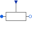VariableResistor Modelica.Electrical.MultiPhase.Basic Ideal linear electrical resistors with variable resistance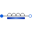SaturatingInductor Modelica.Electrical.MultiPhase.Basic Simple model of inductors with saturation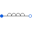Inductor Modelica.Electrical.MultiPhase.Basic Ideal linear electrical inductors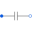Capacitor Modelica.Electrical.MultiPhase.Basic Ideal linear electrical capacitors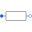Conductor Modelica.Electrical.MultiPhase.Basic Ideal linear electrical conductorsResistor Modelica.Electrical.MultiPhase.Basic Ideal linear electrical resistors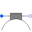Brush Modelica.Electrical.Machines.Losses.InductionMachines Model considering voltage drop of carbon brushes